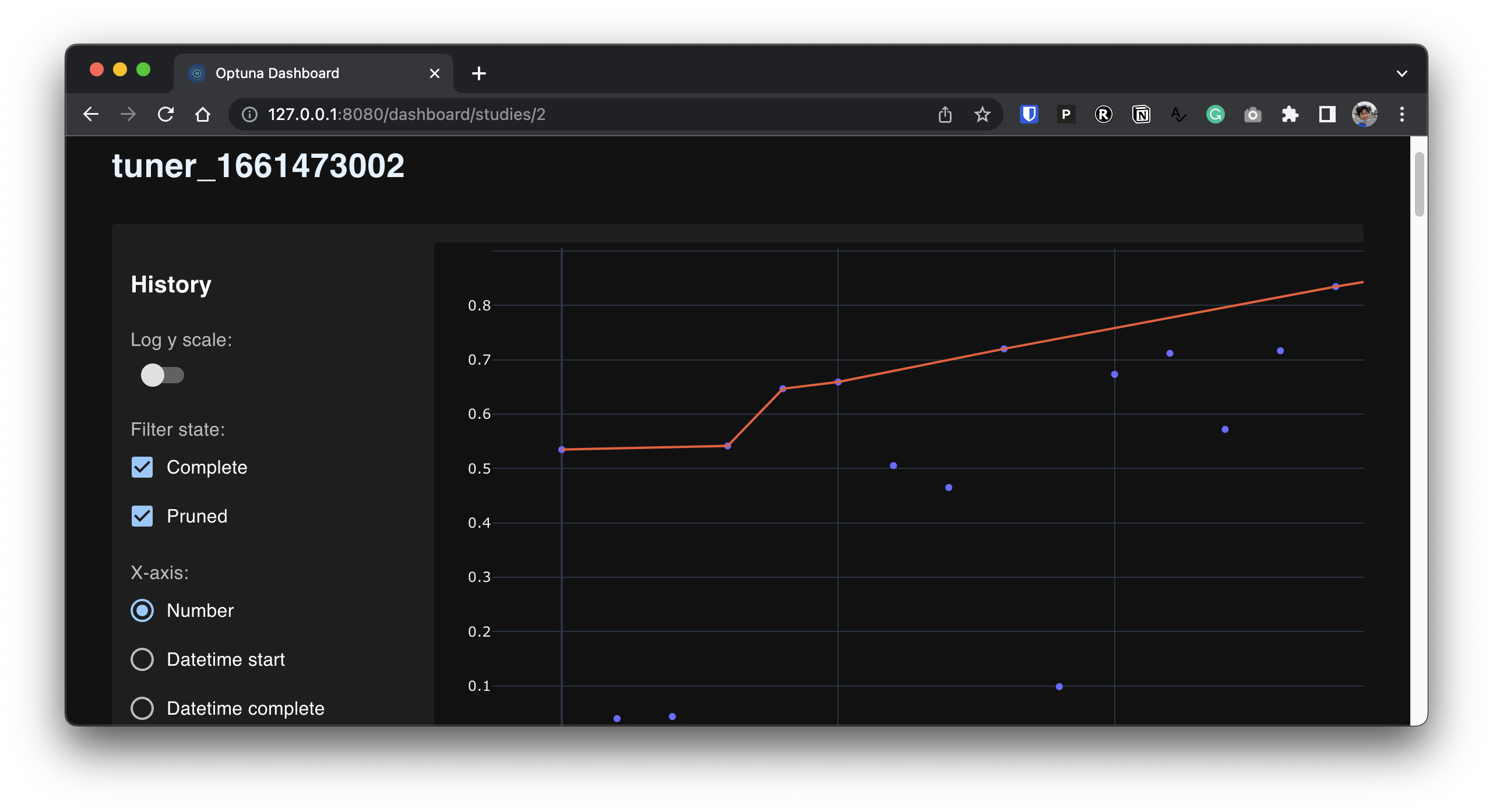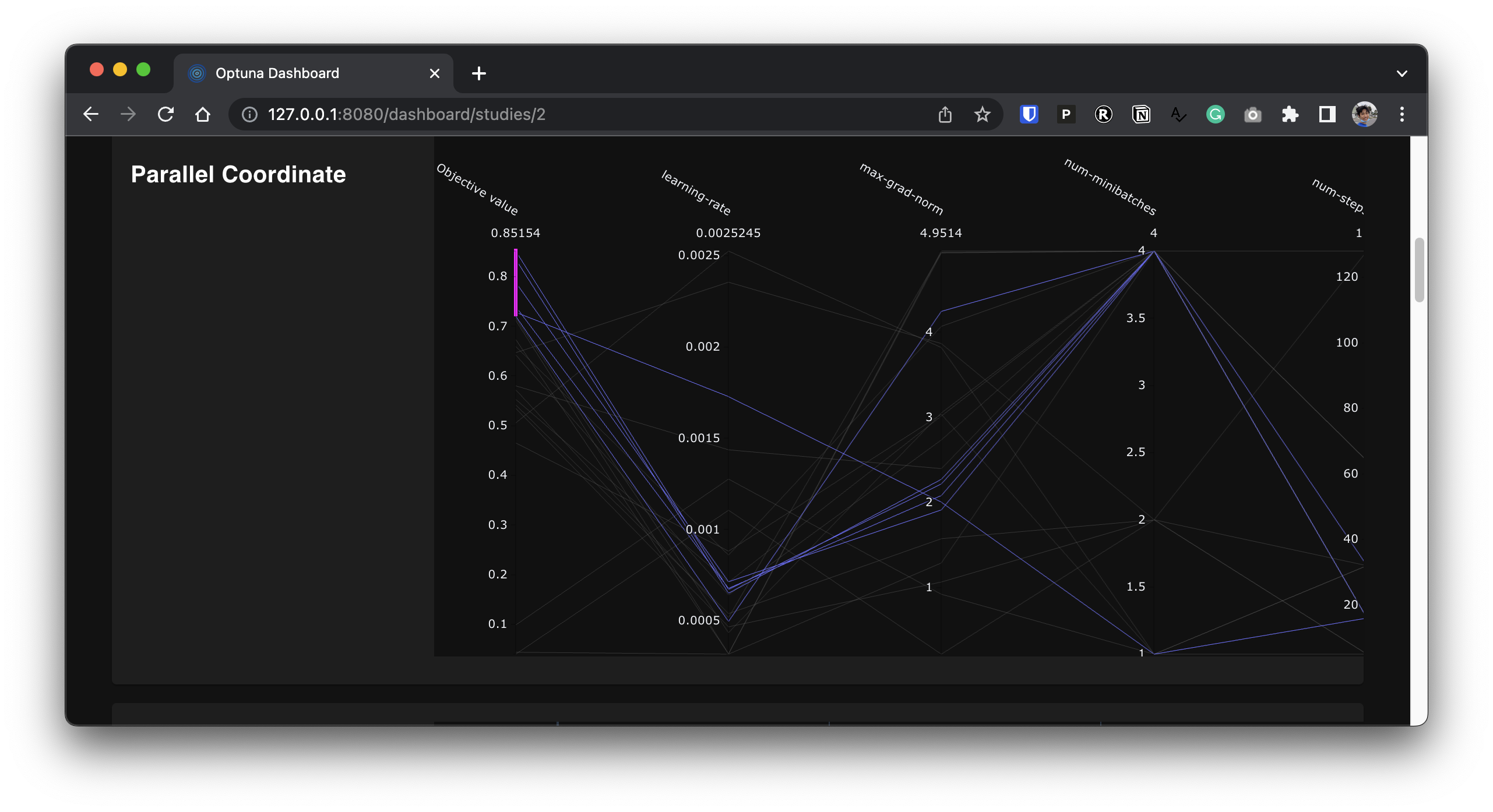# Hyperparameter Tuning

CleanRL comes with a lightweight hyperparameter tuning utility Tuner, with a primary purpose of helping researchers find a single set of hyperparameters that works well with multiple tasks of similar type (e.g., one set of parameters for MuJoCo, another set for Atari). We try to avoid tuning hyperparameters per task, which is usually adhoc and do not generalize.

## Get started

Consider the tuner_example.py in the CleanRL's root directory, which has the following content:

tuner_example.py
import optuna

from cleanrl_utils.tuner import Tuner

tuner = Tuner(
script="cleanrl/ppo.py",
metric="charts/episodic_return",
metric_last_n_average_window=50,
direction="maximize",
aggregation_type="average",
target_scores={
"CartPole-v1": [0, 500],
"Acrobot-v1": [-500, 0],
},
params_fn=lambda trial: {
"learning-rate": trial.suggest_loguniform("learning-rate", 0.0003, 0.003),
"num-minibatches": trial.suggest_categorical("num-minibatches", [1, 2, 4]),
"update-epochs": trial.suggest_categorical("update-epochs", [1, 2, 4, 8]),
"num-steps": trial.suggest_categorical("num-steps", [5, 16, 32, 64, 128]),
"vf-coef": trial.suggest_uniform("vf-coef", 0, 5),
"total-timesteps": 100000,
"num-envs": 16,
},
pruner=optuna.pruners.MedianPruner(n_startup_trials=5),
sampler=optuna.samplers.TPESampler(),
)
tuner.tune(
num_trials=100,
num_seeds=3,
)


You can run the tuner with the following command:

poetry install -E optuna
python tuner_example.py


Often it is useful to find a single set of hyper parameters that work well with multiple environments, but this is challenging because each environment may have different reward scales, so we need to normalize the reward scales.

This is where target_scores comes in. We can use it to specify an upper and lower threshold of rewards for each environment (they don't have to be exact boundaries and can be just ballpark estimates). For example, if we want to find a set of hyper parameters that work well with CartPole-v1 and Acrobot-v1, we can set the target_scores and aggregation_type as follows:

tuner = Tuner(
...,
target_scores={
"CartPole-v1": [0, 500],
"Acrobot-v1": [-500, 0],
}
aggregation_type="average",
)


Here is what happened when running python tuner_example.py:

1. The tuner_example.py launches num_trials=100 trials to find the best single set of hyperparameters for CartPole-v1 and Acrobot-v1 in script="cleanrl/ppo.py".
2. Each trial samples a set of hyperparameters from the params_fn to run num_seeds=3 experiments with different random seeds, mitigating the impact of randomness on the results.
• In each experiment, tuner_example.py averages the last metric_last_n_average_window=50 reported metric="charts/episodic_return" to a number $$x_i$$ and calculate a normalized score $$z_i$$ according to the target_scores. In this case, $$z_{i_0} = (x_{i_0} - 0) / (500 - 0)$$ and $$z_{i_1} = (x_{i_1} - -500) / (0 - -500)$$. Then the tuner will aggregation_type="average" the two scores to get the normalized score $$z_i = (z_{i_0} + z_{i_1}) / 2$$.
3. Each trial then averages the normalized scores $$z_i$$ of the num_seeds=3 experiments to a number $$z$$ and the tuner optimizes $$z$$ according direction="maximize".

Note that we are using 3 random seeds for each environment in ["CartPole-v1","Acrobot-v1"], totalling 2*3=6 experiments per trial.

Info

When optimizing Atari games, you can put the human normalized scores in target_scores (Mnih et al., 2015, Extended Data Table 2)1, as done in the following example. The first number for each environment is the score obtained by random play and the second number is the score obtained by professional game testers. Note here we using aggregation_type="median", this means we will optimize for the median of the human normalized scores averaged over the num_seeds=3 experiments for each trial (pay close attention to the phrasing).

tuner = Tuner(
script="cleanrl/ppo_atari_envpool.py",
metric="charts/episodic_return",
metric_last_n_average_window=50,
direction="maximize",
aggregation_type="median",
target_scores={
"Alien-v5": [227.8, 6875],
"Amidar-v5": [5.8, 1676],
'Assault-v5': (222.4, 1496),
'Asterix-v5': (210.0, 8503),
'Asteroids-v5': (719.1, 13157),
...
},
num_seeds=3,
...
)


## Visualization

Running python tuner_example.py will create a sqlite database containing all of the hyperparameter trials in ./cleanrl_hpopt.db We can use optuna-dashboard to visualize the process.

poetry run optuna-dashboard sqlite:///cleanrl_hpopt.dbIn the panel above, the y-axis shows the normalized score of the two environments (CartPole-v1 and Acrobot-v1) and the x-axis shows the number of trials.The panel above shows the parallel coordinates plot of the normalized score — for example, we can see having a lower learning rate and a higher number of minibatches is a good choice.

Info

You can use a different database by passing Tuner(..., storage="mysql://root@localhost/example") to use a more persistent storage.

## Work w/o knowing the reward scales

What if we don't know the reward scales for the environments? We can optionally set the value target_scores to None, but this will only work with one environment at a time because it's difficult to optimize without knowing the scale of the rewards in each environment.

tuner_example.py
import optuna

from cleanrl_utils.tuner import Tuner

tuner = Tuner(
script="cleanrl/ppo.py",
metric="charts/episodic_return",
metric_last_n_average_window=50,
direction="maximize",
aggregation_type="average",
target_scores={
"CartPole-v1": None,
},
params_fn=lambda trial: {
"learning-rate": trial.suggest_loguniform("learning-rate", 0.0003, 0.003),
"num-minibatches": trial.suggest_categorical("num-minibatches", [1, 2, 4]),
"update-epochs": trial.suggest_categorical("update-epochs", [1, 2, 4, 8]),
"num-steps": trial.suggest_categorical("num-steps", [5, 16, 32, 64, 128]),
"vf-coef": trial.suggest_uniform("vf-coef", 0, 5),
"total-timesteps": 100000,
"num-envs": 16,
},
pruner=optuna.pruners.MedianPruner(n_startup_trials=5),
sampler=optuna.samplers.TPESampler(),
)
tuner.tune(
num_trials=100,
num_seeds=3,
)


In the example above, we will set the normalized score $$z_i$$ to be the average of the last metric_last_n_average_window=50 reported metric="charts/episodic_return" for CartPole-v1.

## Work w/ pruners and samplers

You can use Tuner with any pruner from optuna to prune less promising experiments or samplers to sample new hyperparameters. If you don't specify them explicitly, the script will use the default ones.

tuner_example.py
import optuna

from cleanrl_utils.tuner import Tuner

tuner = Tuner(
script="cleanrl/ppo.py",
metric="charts/episodic_return",
metric_last_n_average_window=50,
direction="maximize",
aggregation_type="average",
target_scores={
"CartPole-v1": None,
},
params_fn=lambda trial: {
"learning-rate": trial.suggest_loguniform("learning-rate", 0.0003, 0.003),
"num-minibatches": trial.suggest_categorical("num-minibatches", [1, 2, 4]),
"update-epochs": trial.suggest_categorical("update-epochs", [1, 2, 4, 8]),
"num-steps": trial.suggest_categorical("num-steps", [5, 16, 32, 64, 128]),
"vf-coef": trial.suggest_uniform("vf-coef", 0, 5),
"total-timesteps": 100000,
"num-envs": 16,
},
pruner=optuna.pruners.MedianPruner(n_startup_trials=5),
sampler=optuna.samplers.TPESampler(),
)
tuner.tune(
num_trials=100,
num_seeds=3,
)


## Track experiments w/ Weights and Biases

The Tuner can track all the experiments into Weights and Biases to help you visualize the progress of the tuning.

tuner_example.py
import optuna

from cleanrl_utils.tuner import Tuner

tuner = Tuner(
script="cleanrl/ppo.py",
metric="charts/episodic_return",
metric_last_n_average_window=50,
direction="maximize",
aggregation_type="average",
target_scores={
"CartPole-v1": None,
},
params_fn=lambda trial: {
"learning-rate": trial.suggest_loguniform("learning-rate", 0.0003, 0.003),
"num-minibatches": trial.suggest_categorical("num-minibatches", [1, 2, 4]),
"update-epochs": trial.suggest_categorical("update-epochs", [1, 2, 4, 8]),
"num-steps": trial.suggest_categorical("num-steps", [5, 16, 32, 64, 128]),
"vf-coef": trial.suggest_uniform("vf-coef", 0, 5),
"total-timesteps": 100000,
"num-envs": 16,
},
pruner=optuna.pruners.MedianPruner(n_startup_trials=5),
sampler=optuna.samplers.TPESampler(),
wandb_kwargs={"project": "cleanrl"},
)
tuner.tune(
num_trials=100,
num_seeds=3,
)


1. Mnih, V., Kavukcuoglu, K., Silver, D. et al. Human-level control through deep reinforcement learning. Nature 518, 529–533 (2015). https://doi.org/10.1038/nature14236